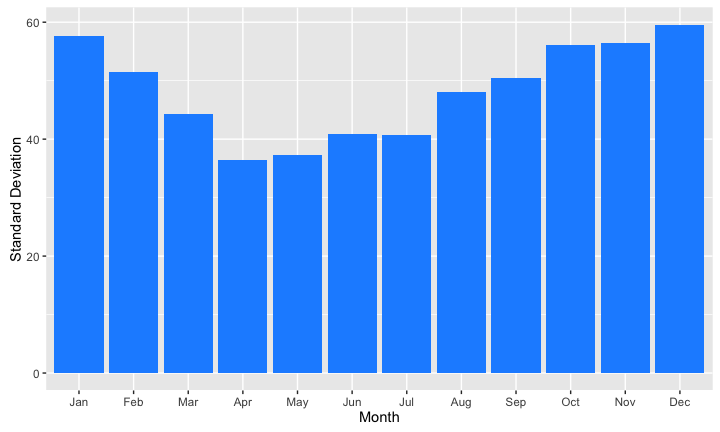## Overview

• Pipelines
• Creating Plots
• Basic Time Series

## 💧 One data set we'll use is `rainfall.RData`…

📥 Get it from the Moodle site in section headed Chris Brunsdon's Lectures and Materials Week 2.
```load('rainfall.RData')
```# A tibble: 6 × 9
Station Elevation Easting Northing   Lat  Long    County
<chr>     <int>   <dbl>    <dbl> <dbl> <dbl>     <chr>
1      Athboy        87  270400   261700 53.60 -6.93     Meath
2 Foulksmills        71  284100   118400 52.30 -6.77   Wexford
3   Mullingar       112  241780   247765 53.47 -7.37 Westmeath
4     Portlaw         8  246600   115200 52.28 -7.31 Waterford
5    Rathdrum       131  319700   186000 52.91 -6.22   Wicklow
6 Strokestown        49  194500   279100 53.75 -8.10 Roscommon
# ... with 2 more variables: Abbreviation <chr>, Source <chr>```

## This packages all of the rainfall information

`head(rain)`
```# A tibble: 6 × 4
Year Month Rainfall Station
<dbl> <ord>    <dbl>   <chr>
1  1850   Jan    169.0  Ardara
2  1851   Jan    236.4  Ardara
3  1852   Jan    249.7  Ardara
4  1853   Jan    209.1  Ardara
5  1854   Jan    188.5  Ardara
6  1855   Jan     32.3  Ardara```

• Its been converted to an R binary data file for convenience.
• There are no missing values.
• Data runs from 1850 to 2014 by month.
• 👍🏻Thanks to Conor Murphy and Simon Noone for supplying.

## 🎁 A new package `dplyr`

```library(dplyr); library(tidyverse)
rain %>% group_by(Station) %>%
summarise(mrain=mean(Rainfall))  -> rain_summary
```# A tibble: 6 × 2
Station     mrain
<chr>     <dbl>
1     Ardara 140.36753
2     Armagh  68.32096
3     Athboy  74.74356
4    Belfast  87.10995
5       Birr  70.83498
6 Cappoquinn 121.22455```

• The `%>%` command acts as a 'pipeline'.
• `x %>% f(y)` is the same as `f(x,y)`.
• `x %>% f1(y) %>% f2(z)` is the same as `f2(f1(x,y),z)` but easier to read.

## 📆 Group by month

```rain %>% group_by(Month) %>%
summarise(mrain=mean(Rainfall)) -> rain_months
```# A tibble: 6 × 2
Month     mrain
<ord>     <dbl>
1   Jan 112.64355
2   Feb  83.24975
3   Mar  79.53280
4   Apr  68.74165
5   May  71.31769
6   Jun  72.74104```

`summarise` applies a summary function to each group and creates a new data frame with one entry for each group. Any R summary function can be used - eg `median`, `sd`, `max` or a user defined function.

## 📊 Basic graphic investigation

```library(ggplot2)
ggplot(aes(x=Month,y=mrain),data=rain_months) + geom_bar(stat='identity') + labs(y='Mean Rainfall')```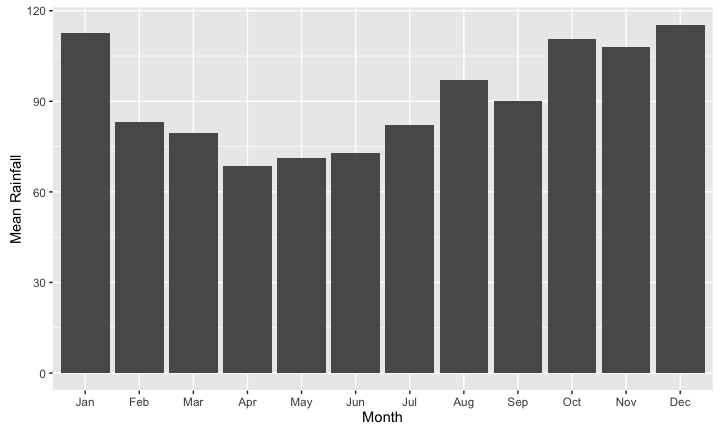## 📈 Also for yearly data

```rain %>% group_by(Year) %>%
summarise(total_rain=sum(Rainfall)) -> rain_years
ggplot(aes(x=Year,y=total_rain),data=rain_years) + geom_line(stat='identity') + labs(y='Mean Annual Rainfall')```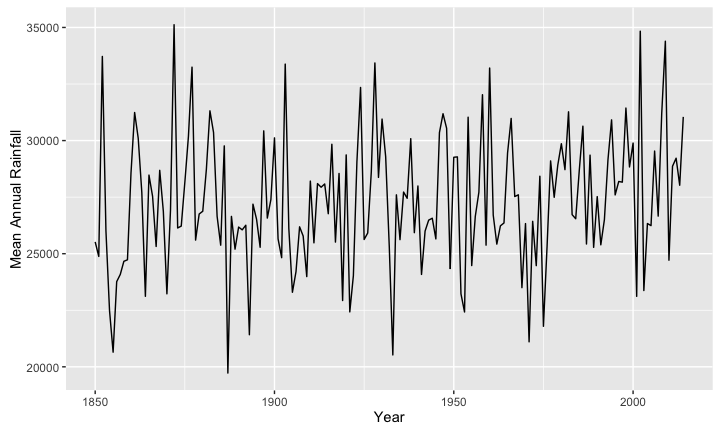## ✂️ Filter for individual stations using `filter`

```rain %>% group_by(Year) %>%
filter(Station=='Strokestown') %>%
summarise(total_rain=sum(Rainfall)) -> rain_years_str
ggplot(aes(x=Year,y=total_rain),data=rain_years_str) + geom_line(stat='identity') +
labs(y='Mean Annual Rainfall') -> strokes
strokes```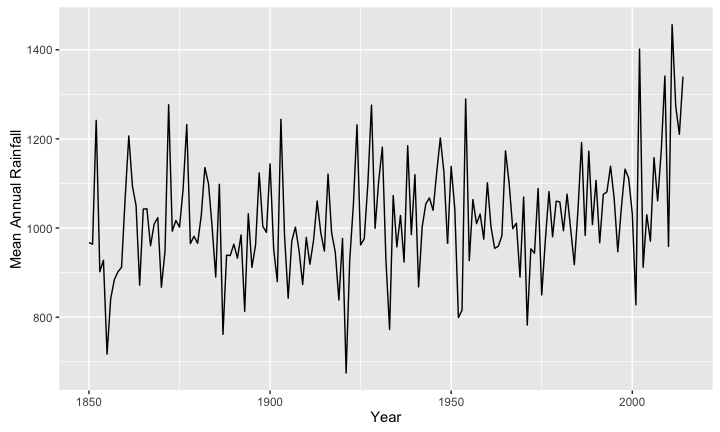## Is there a trend here?

`strokes + geom_smooth()`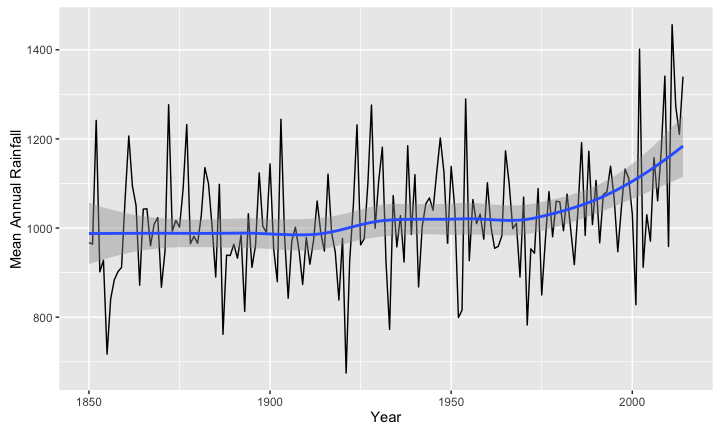## 🔢 Multiple groupings - eg average by month/station combination

```rain %>% group_by(Month,Station) %>%
summarise(mean_rain=mean(Rainfall)) -> rain_season_station
```Source: local data frame [6 x 3]
Groups: Month 

Month    Station mean_rain
<ord>      <chr>     <dbl>
1   Jan     Ardara 174.82606
2   Jan     Armagh  74.57242
3   Jan     Athboy  84.94759
4   Jan    Belfast 101.20718
5   Jan       Birr  79.92074
6   Jan Cappoquinn 153.97159```

Note that `with` is implicit in the `dplyr` commands such as `filter` and `summarise`

## Re-arrange using `reshape2` package

```library(reshape2)
```                 Jan       Feb       Mar      Apr      May       Jun
Ardara     174.82606 126.82303 123.02000 98.79333 96.90727 105.24061
Armagh      74.57242  55.97182  56.48879 53.67030 59.23182  62.72939
Athboy      84.94759  62.62133  62.44944 58.97874 62.16260  68.11460
Belfast    101.20718  74.50206  73.10221 65.90492 69.23426  74.48525
Birr        79.92074  57.88501  58.42056 54.07187 60.25831  61.96445
Cappoquinn 153.97159 117.77099 110.02890 94.00365 95.81437  94.86357
Jul       Aug       Sep       Oct       Nov       Dec
Ardara     123.70485 145.24788 152.80727 174.44788 176.45030 186.14182
Armagh      72.50636  81.92182  69.02576  80.94242  73.73121  79.05939
Athboy      76.28662  88.84710  76.35077  88.14627  80.95767  87.05997
Belfast     87.70003 102.44499  87.11622 106.35394 100.31760 102.95073
Birr        75.10084  86.75669  72.21722  83.80810  77.87266  81.74334
Cappoquinn 104.09372 125.42245 116.04466 146.70658 141.10012 154.87402```

• Now results organised into 2D array

## … which makes a useful heatmap

```library(reshape2)
rain_season_station %>% acast(Station~Month) %>% heatmap(Colv=NA)```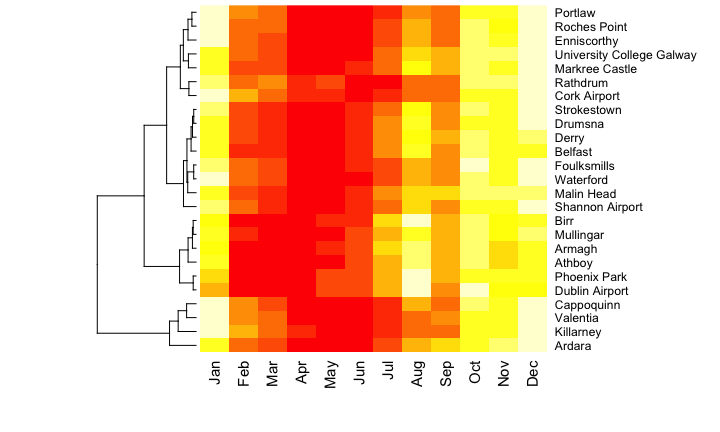## Why `%>%` is useful here

This code takes the `rain` data frame and runs through the steps to aggregated, reshapeand compute the heat map:

```rain %>% group_by(Month,Station) %>% summarise(mean_rain=mean(Rainfall)) %>%
acast(Station~Month) %>%
heatmap(Colv=NA)```

So does this code, in 'traditional' R format:

```  heatmap(acast(summarise(group_by(rain,Month,Station),
mean_rain=mean(Rainfall)),Station~Month),Colv=NA)```

Which do you think is clearer?

## Multiple summary stats are also possible

```rain %>% group_by(Month,Station) %>%
summarise(mean_rain=mean(Rainfall),sd_rain=sd(Rainfall)) -> rain_mnsd
```Source: local data frame [6 x 4]
Groups: Month 

Month    Station mean_rain  sd_rain
<ord>      <chr>     <dbl>    <dbl>
1   Jan     Ardara 174.82606 64.59834
2   Jan     Armagh  74.57242 28.91264
3   Jan     Athboy  84.94759 32.84887
4   Jan    Belfast 101.20718 40.60078
5   Jan       Birr  79.92074 33.74263
6   Jan Cappoquinn 153.97159 66.33995```

## `mutate` - Create new variables in a data frame

```rain %>% group_by(Month) %>%
summarise(mean_rain=mean(Rainfall),sd_rain=sd(Rainfall)) %>%
mutate(cv_rain=100 * sd_rain/mean_rain)  -> rain_mnsdcv
```# A tibble: 6 × 4
Month mean_rain  sd_rain  cv_rain
<ord>     <dbl>    <dbl>    <dbl>
1   Jan 112.64355 57.56599 51.10456
2   Feb  83.24975 51.45153 61.80382
3   Mar  79.53280 44.33714 55.74699
4   Apr  68.74165 36.39073 52.93841
5   May  71.31769 37.20539 52.16853
6   Jun  72.74104 40.92818 56.26560```

• 📖 CV: Coefficient of variation - standard deviation as a percentage of mean.
• Shows relative variability of rainfall

## Relative variability visualised…

`ggplot(aes(x=Month,y=cv_rain), data=rain_mnsdcv) + geom_bar(stat='identity') + labs(y='Coefficient of Variation')`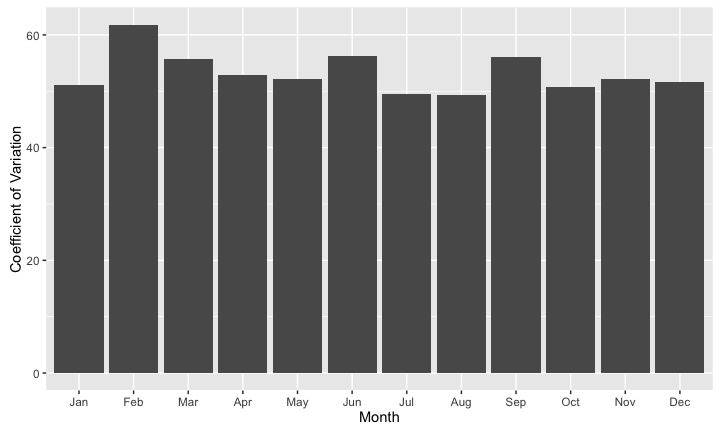## … and absolute variability

`ggplot(aes(x=Month,y=sd_rain), data=rain_mnsdcv) + geom_bar(stat='identity',fill='dodgerblue') + labs(y='Standard Deviation')`